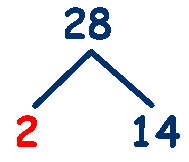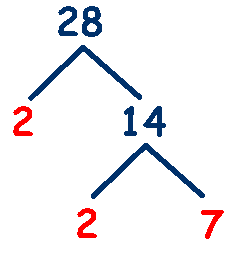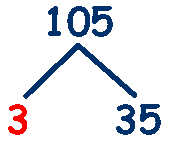# Prime Numbers

## Prime numbers are numbers that can only be divided by themselves and 1, in other words they only have two factors .... 1 and themselves.

Look at the list of factors below and identify which numbers are prime.

So the first prime numbers are 2,3,5,7 and 11

Notice that 2 is the only even prime number - all the others are odd.

## How do we find out which prime factors make up a number?

There are 2 methods

## Method 1: To find the prime factors of 28.

Begin with the smallest prime number that will divide exactly into the number - which is 2 (2 divides into all even numbers) so we can write .... 28 = 2 x 14

Next find the smallest prime that will divide exactly into 14 - which is again 2, so 14 = 2 x 7
so we can write .... 28 = 2 x 2 x 7

All of these numbers are prime - so we are finished .... the prime factors of 28 are 2, 2 and 7

### Lets find the prime factors of 105

Smallest prime that will divide exactly into 105 is 3 ... 105 = 3 x 35

Smallest prime that will divide exactly into 35 is 5 ... 35 = 5 x 7

So we can write .... 105 = 3 x 5 x 7 ... the prime factors of 105 are 3, 5 and 7

## Method 2: To find the prime factors of 28 using a factor tree.

Using the factor tree method is essentially the same as method 1,
but you are representing each step in a more visual way.

The first step is to find the smallest prime number that will divide exactly into 28. As 28 is an even number, the smallest prime will be 2.

28 = 2 x 14 - Draw 2 lines (like branches of a tree) from the number 28 and put the 2 on the left and the 14 on the right.

Next we have to find the smallest prime number that will divide exactly into 14. As 14 is an even number, the smallest prime will be 2.

14 = 2 x 7 - Draw 2 lines from the number 14 and put the 2 on the left and the 7 on the right.

All of these numbers are prime - so we are finished ....

### Lets find the prime factors of 105.

The first step is to find the smallest prime number that will divide exactly into 105.
The smallest prime is 3.

105 = 3 x 35 - Draw 2 lines from the number 105 and put the 3 on the left and the 35 on the right.

The next step is to find the smallest prime number that will divide exactly into 35.
The smallest prime is 5.

35 = 5 x 7 - Draw 2 lines from the number 35 and put the 5 on the left and the 7 on the right.

All of these numbers are prime - so we are finished ....

### The prime factors of 28 are 3, 5 and 7.________________With the exception of 5, there are no prime numbers ending in 5 and with the exception of 2, there are no even prime numbers. So apart from 2 and 5, all prime numbers end in 1, 3, 7 or 9.

There are prizes for finding the next largest prime number, in 2009, \$100,000 was awarded for finding a prime number with over 10 million digits. The next prize on offer is for the first prime number to exceed 100,000,000 digits. Well, you've made a start!

Here is a list of the First 1000 Prime Numbers

A worksheet on How to find prime factors
from Mathsblog

A 100 number square to find prime numbers